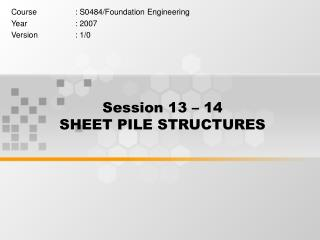DownloadDownload PresentationSession 13 – 14 SHEET PILE STRUCTURES

# Session 13 – 14 SHEET PILE STRUCTURES

Télécharger la présentation## Session 13 – 14 SHEET PILE STRUCTURES

- - - - - - - - - - - - - - - - - - - - - - - - - - - E N D - - - - - - - - - - - - - - - - - - - - - - - - - - -
##### Presentation Transcript

1. Session 13 – 14 SHEET PILE STRUCTURES Course : S0484/Foundation Engineering Year : 2007 Version : 1/0

2. SHEET PILE STRUCTURES Topic: • General • Types of Sheet Pile • Lateral Pressure Diagram • Cantilever Sheet Pile

3. SHEET PILE

4. GENERAL Connected or semi-connected sheet piles are often used to build continuous walls to retain the lateral pressure caused by soil or external load. In contrast to the construction of other types of retaining wall, the building of sheet pile walls do not usually require dewatering the site. Sheet piles are also used for some temporary structures, such as braced cut.

5. SHEET PILE TYPES CANTILEVER SHEET PILE

6. ANCHORED SHEET PILE SHEET PILE TYPES Free Earth Support

7. ANCHORED SHEET PILE SHEET PILE TYPES Fixed Earth Support

8. ANCHORED SHEET PILE SHEET PILE TYPES anchor plate or beam

9. ANCHORED SHEET PILE SHEET PILE TYPES tie back vertical anchor pile

10. ANCHORED SHEET PILE SHEET PILE TYPES anchor beam with batter piles

11. LATERAL EARTH PRESSURE DIAGRAM

12. LATERAL EARTH PRESSURE DIAGRAM

13. LATERAL EARTH PRESSURE DIAGRAM Fixed Earth Support

14. LATERAL EARTH PRESSURE DIAGRAM Free Earth Support

15. LATERAL EARTH PRESSURE DIAGRAM Free Earth Support

16. CALCULATION STEPSCANTILEVER SHEET PILE - SAND

17. CALCULATION STEPSCANTILEVER SHEET PILE - SAND 1. Determine the value of Ka and Kp 2. Calculate p1and p2 with L1 and L2 are known 3. Calculate L3 4. Calculate the resultant of the area ACDE (P) 5. Determine the z (the center of pressure for the area ACDE)

18. CALCULATION STEPSCANTILEVER SHEET PILE - SAND 6. Calculate p5 7. Calculate A1, A2, A3, A4

19. CALCULATION STEPSCANTILEVER SHEET PILE - SAND 8. Determine L4 9. Calculate p4 10. Calculate p3 11. Calculate L5 12. Draw the pressure distribution diagram 13. Obtain the theoretical depth ; D = L3 + L4 The actual depth of penetration is increased by about 20% - 30% 14. Calculate the maximum bending moment

20. EXAMPLECANTILEVER SHEET PILE - SAND L1 = 2 m L2 = 3 m GWL • d = 15.9 kN/m3 • t = 19.33 kN/m3 • = 32o c = 0 kPa D Determine the penetration depth (D) and dimension of sheet pile

21. EXAMPLECANTILEVER SHEET PILE - SAND • Step 1 (determine the value of ka and kp) • Step 2 (calculate p1 and p2) • Step 3 (Calculate L3) kPa kPa m

22. EXAMPLECANTILEVER SHEET PILE - SAND • Step 4 (calculate P) • Step 5 (calculate z) • Step 6 (calculate p5) kN/m m kN/m2

23. EXAMPLECANTILEVER SHEET PILE - SAND • Step 7 (calculate A1 – A4)

24. EXAMPLECANTILEVER SHEET PILE - SAND • Step 8 (determine L4) • Step 9 (calculate p4) • Step 10 (calculate p3) L4 4.8 m kPa kPa

25. EXAMPLECANTILEVER SHEET PILE - SAND • Step 11 (Calculate L5) • Step 12 Draw the pressure distribution diagram • Step 13 (the penetration dept of sheet pile) • Theoretical = 0.66 + 4.8 = 5.46 m • Actual = 1.3 (L3+L4) =7.1 m m

26. EXAMPLECANTILEVER SHEET PILE - SAND

27. EXAMPLECANTILEVER SHEET PILE - SAND Dimension of Sheet Pile m kN.m

28. CALCULATION STEPSCANTILEVER SHEET PILE - CLAY

29. CALCULATION STEPSCANTILEVER SHEET PILE - CLAY 1. Determine the value of Ka and Kp In case of saturated soft clay with internal friction angle () = 0, we got Ka = Kp = 1 2. Calculate p1and p2 with L1 and L2 are known 3. Calculate the resultant of the area ACDE (P1) and z1 (the center of pressure for the area ACDE)

30. CALCULATION STEPSCANTILEVER SHEET PILE - CLAY 4. Calculate the theoretical penetration depth of sheet pile (D) 5. Calculate L4 6. Calculate p6 and p7 7. Obtain the actual penetration depth of sheet pile Dactual = (1.4 – 1.6) x Dtheoretical

31. CALCULATION STEPSCANTILEVER SHEET PILE - CLAY 8. Calculate the maximum bending moment

32. EXAMPLECANTILEVER SHEET PILE - CLAY L1 = 2 m L2 = 3 m sand GWL • d = 15.9 kN/m3 • t = 19.33 kN/m3 • = 32o c = 0 kPa Clay D cu = 47 kPa  = 0 o Determine the penetration depth (D) and dimension of sheet pile

33. EXAMPLECANTILEVER SHEET PILE - CLAY • Step 1 (Determine ka and kp) • Step 2 (calculate p1 and p2) • Step 3 (calculate P1and z1) kPa kPa kN/m m

34. EXAMPLECANTILEVER SHEET PILE - CLAY • Step 4 (obtain Dtheoretical) • Step 5 (calculate L4) D = 2.13 m L4 = 2.13 m

35. EXAMPLECANTILEVER SHEET PILE - CLAY • Step 6 (calculate p6 and p7) • Step 7 (draw the lateral diagram) • Step 8 (Obtain Dactual) kN/m2 kN/m2 Dactual = 1.5 x Dtheorical = 1.5 x 2.13 = 3.2 m

36. EXAMPLECANTILEVER SHEET PILE - CLAY • Calculation of moment